# 归并排序 详解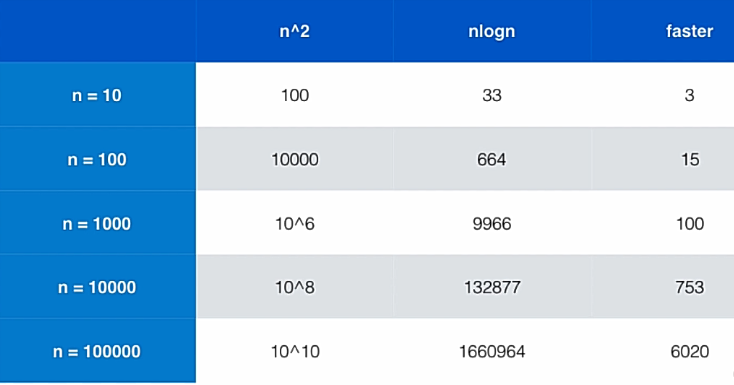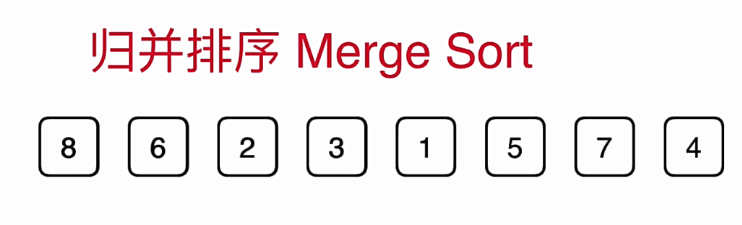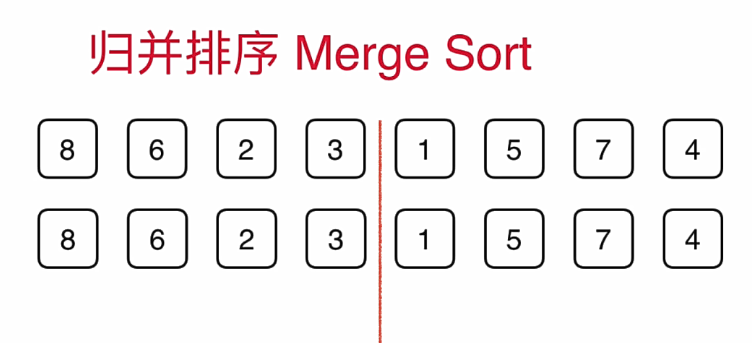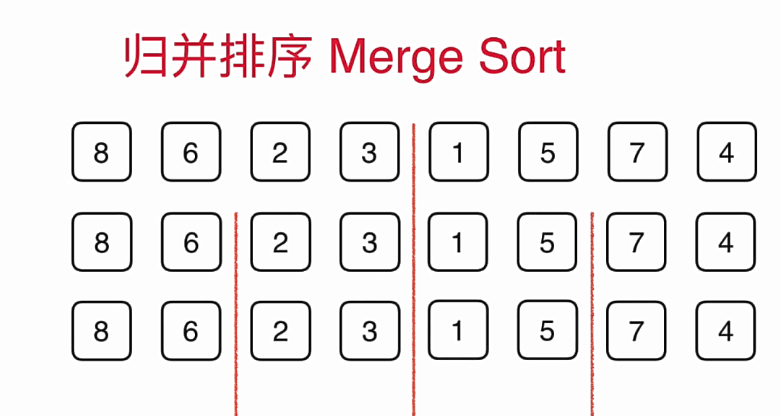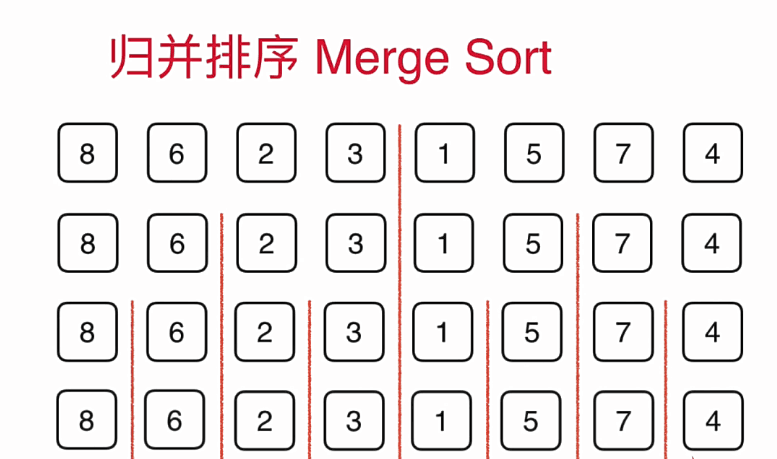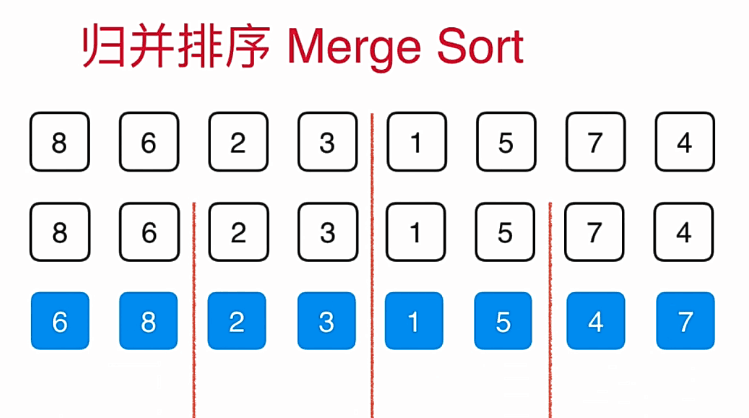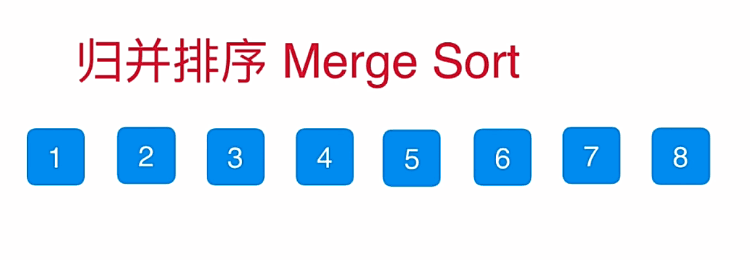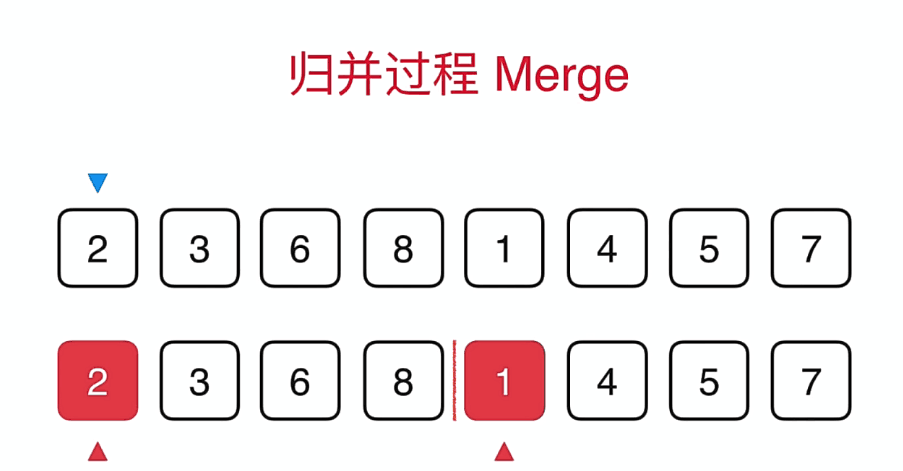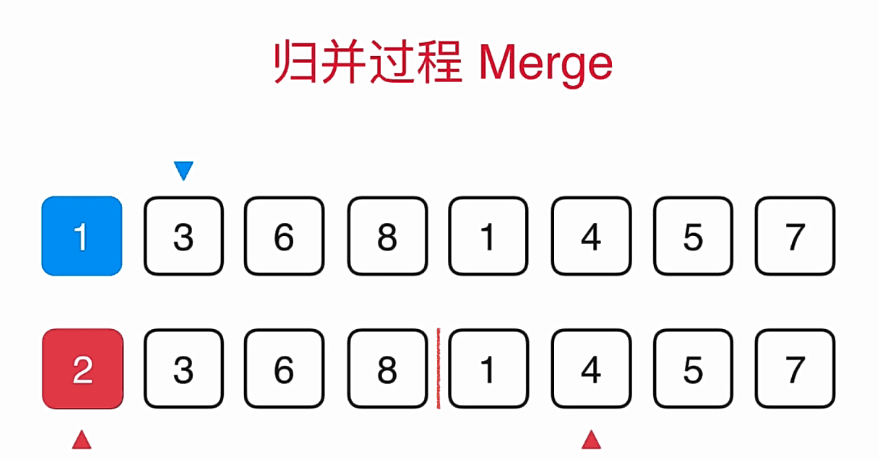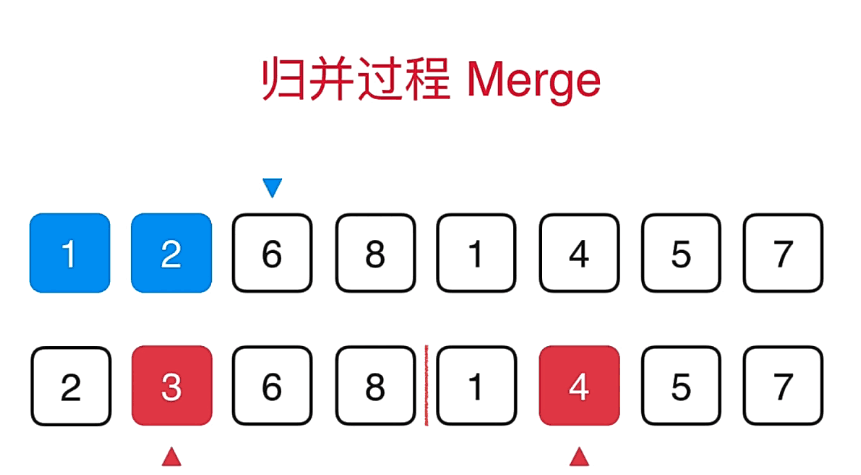#include<cstdio>
#include<iostream>
#include<algorithm>
#include<cstring>
using namespace std;

void merge(int a[],int l,int r,int mid)
{
int aux[r-l+1],i,j,k;

for(k=l;k<=r;k++)
aux[k-l]=a[k];

i=l;
j=mid+1;
for(k=l;k<=r;k++)
{
if(i>mid)
{
a[k]=aux[j-l];
j++;
}
else if(j>r)
{
a[k]=aux[i-l];
i++;
}
else if(aux[i-l]>aux[j-l])
{
a[k]=aux[j-l];
j++;
}
else
{
a[k]=aux[i-l];
i++;
}

}

}

void merge_sort(int a[],int l,int r)
{
if(l>=r)
return ;

int mid=(l+r)/2;

merge_sort(a,l,mid);
merge_sort(a,mid+1,r);
merge(a,l,r,mid);

}

void mergesort(int a[],int l,int r)
{
merge_sort(a,l,r-1);
}

int main()
{
int a,n,i;
scanf("%d",&n);

for(i=0;i<n;i++)
scanf("%d",&a[i]);

mergesort(a,0,n);

for(i=0;i<n;i++)
printf("%d ",a[i]);

return 0;
} 

12-09420
10-3124
09-25188
11-11890
09-10197
03-07328
05-21156
07-201884
12-07243
06-07441
10-043075
01-062568
05-17315
08-07525
05-10136
04-15171
01-061031
06-15231

### “相关推荐”对你有帮助么？

•非常没帮助
•没帮助
•一般
•有帮助
•非常有帮助被折叠的  条评论 为什么被折叠?到【灌水乐园】发言¥2 ¥4 ¥6 ¥10 ¥20余额支付 (余额：-- )扫码支付获取中扫码支付点击重新获取扫码支付1.余额是钱包充值的虚拟货币，按照1:1的比例进行支付金额的抵扣。
2.余额无法直接购买下载，可以购买VIP、C币套餐、付费专栏及课程。余额充值